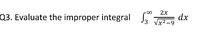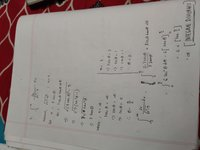# Is my solution for this particular improper integral question correct?

#### math_N00b

##### New member
The question:I looked up an online improper integral calculator which returned the same result that the integral diverges but it solved in a different way, my only question is, is this way of solving improper integrals using trig substitutions correct? I just need confirmations because my course instructor also did other improper integral probelms in another way using limits, should I resort to that instead?

#### pka

##### Elite Member
There is no one correct way to do these problems.
Is it true that the derivative of $$2\sqrt{x^2-9}$$ is $$\dfrac{2x}{\sqrt{x^2-9}}~?$$
If so what is $$\mathop {\lim }\limits_{x \to \infty } 2\sqrt {{x^2} - 9} ~?$$
As I see the question, the above answers it. How one gets there is really unimportant.

•math_N00b and topsquark

#### Dr.Peterson

##### Elite Member
I used an entirely different substitution, which was easier (I think), but no more valid than yours. That, as pka said, is unimportant.

On the other hand, you can't really say, "when x = ∞ ..."! Technically, you are taking a limit there, without saying so; it would be more proper to make it explicit. This depends on the nature of your course, ultimately; in effect, I did the same thing you did there, because I just wanted to find the answer, not to satisfy a teacher's standards. In some courses, care is expected (as if you were writing a proof), while in others, getting the right answer is enough. And as pka said, if you check it and it works, then it doesn't matter how you got there.

You did do a check, right?

•math_N00b and topsquark

#### Jomo

##### Elite Member
The way you did the integral was fine! I just want to add to your thinking process. Since the derivative of what is under that square root is sitting on top you did not have to do trig substitution. You could have set u = x^2 - 9, du =2xdx and the integral would have been u^-2 du which is simple to solve. The point is don't always assume the integral involves trig substitution whenever you have a^2 + x^2, x^2 - a^2 and a^2-x^2.

•math_N00b